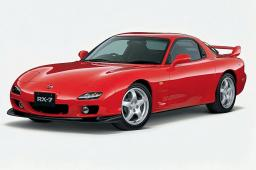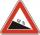# Climb

On the road sign, which informs the climb is 8.7%. The car drive 5 km along this road.

What is the height difference that the car went to?

x =  433.4 m

### Step-by-step explanation:Did you find an error or inaccuracy? Feel free to write us. Thank you!Tips to related online calculators
Pythagorean theorem is the base for the right triangle calculator.

#### You need to know the following knowledge to solve this word math problem:

We encourage you to watch this tutorial video on this math problem:

## Related math problems and questions:

• Traffic signThere is a traffic sign for climbing on the road with an angle of 7%. Calculate at what angle the road rises (falls).
• ClimbRoad has climbing 1:27. How big is a angle corresponds to this climbing?
• Height differenceWhat height difference is overcome if we pass a road 1 km long with a pitch21 per mille?The road sign informs the gradient is 10.9%. Calculate the angle at which the average decreases.The average climb of the road is given by ratio 1:15. By what angle road average climb?Between cities A and B is route 13 km long of stúpanie average 7‰. Calculate the height difference of cities A and B.
• The cable carThe cable car has a length of 3,5 kilometers and an angle of climb of 30 degrees. What is the altitude difference between the Upper and Lower stations?
• RailwaysRailways climb 7.4 ‰. Calculate the height difference between two points on the railway distant 3539 meters.
• Triangle KLBIt is given equilateral triangle ABC. From point L which is the midpoint of the side BC of the triangle it is drwn perpendicular to the side AB. Intersection of perpendicular and the side AB is point K. How many % of the area of the triangle ABC is area o
• RailwayRailway line had on 5.8 km segment climb 9 permille. How many meters track ascent?The angle of a straight road is approximately 12 degrees. Determine the percentage of this road.Side b = 1.5, hypotenuse angle A = 70 degrees, Angle B = 20 degrees. Find its unknown sides length.The cable car is 2610 m long and rises at an angle of 35°. Calculate the height difference between the lower and upper station of the cable car.Cable car rises at an angle 41° and connects the upper and lower station with an altitude difference of 1175 m. How long is the track of cable car?A boy of height 1.7m is standing 30m away from the flagstaff on the same level ground. He observes that the angle of deviation of the top of flagstaff is 30 degrees. Calculate the height of flagstaff.A drive wheel of radius 2 is connected to a drive wheel of radius 1 by a pulley of length 17. What is the distance between the wheel axles?From the church tower's view at the height of 65 m, the top of the house can be seen at a depth angle of alpha = 45° and its bottom at a depth angle of beta = 58°. Calculate the height of the house and its distance from the church.